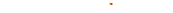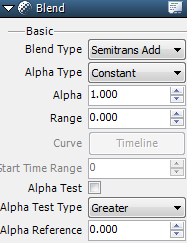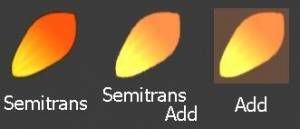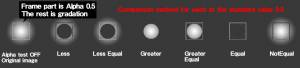####### Blend■Blend Type

 None Draw regular elements Semitrans Draw semitransparent elements based on alpha value Semitrans Add Draw elements by adding transparency（added translucence） Semitrans Sub Draw element by subtracting transparency (subtracted translucence) Add Add drawing element Subtract Subtract drawing element Reverse Invert texture color

Difference between Semitrans, Semitrans Add, and Add■Alpha Type

 Constant Makes the alpha value the opacity level Curve Set the opacity level in Timeline

 Alpha Set the value between 0.0 and 1.0 Curve Create with Timeline when Alpha type is set to “Curve”.

■Random Range

Set the random ranges for Alpha and curve points.

■Start Time Random Range（from Version 1.8）

Set the start timing with a random range when blend settings for curves are being used.

■Alpha Test

Select Comparison Method ON or OFF.

■Comparison Method

 Less When the alpha of the pixels to be drawn is smaller than the standard value then they will be drawn. Less Equal When the alpha of the pixels to be drawn is smaller or the same as the standard value then they will be drawn. Greater When the alpha of the pixels to be drawn is larger than the standard value then they will be drawn. Greater Equal When the alpha of the pixels to be drawn is larger or the same as the standard value then they will be drawn. Equal When the alpha of the pixels to be drawn is larger than the standard value then they will be displayed. Setting only for special expressions. Not Equal When the alpha of the pixels to be drawn is anything other than the standard value then they will be displayed. Setting only for special expressions.

■Standard Values

Set standard values to use in Comparison Method.・Making the alpha value low makes most of the drawing so it can’t be seen and is thus left out which reduces the drawing load.
・Only specific pixels of the texture will be displayed.
This is what alpha testing makes possible.